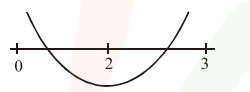`
Question:

$(c-5) x^{2}-2 c x+(c-4)=0, c \neq 5$. Let $S$ be the set

of all integral values of $\mathrm{c}$ for which one root of the equation lies in the interval $(0,2)$ and its other root lies in the interval $(2,3)$. Then the number of elements in $S$ is :

1. 11

2. 18

3. 10

4. 12

Correct Option: 1

Solution:Let $f(x)=(c-5) x^{2}-2 c x+c-4$

$\therefore f(0) f(2)<0$   ...................(1)

$\& f(2) f(3)<0$   ..............(2)

from (1) \& (2)

$(c-4)(c-24)<0$

$\&(c-24)(4 c-49)<0$

$\Rightarrow \frac{49}{4}$\therefore s=\{13,14,15, \ldots .23\}$Number of elements in set$S=11\$